swizec.com

#### Senior Mindset Book

Get promoted, earn a bigger salary, work for top companies

# Week 15: A tutorial on the expressiveness and universality of fold

[This post is part of an ongoing challenge to understand 52 papers in 52 weeks. You can read previous entries, here, or subscribe to be notified of new posts by email]

Fold or foldr is a recursion operator with magical properties commonly used in functional programming. Sometimes known as reduce, inject, or compress it turns a list of values into a single value according to a combining function.

Graham Hutton's 1999 A tutorial on the expressivenes nad universality of fold does a great job of explaining just how magical it is. In a language with first-order tuples and functions any function can be expressed as a fold, it lets you prove programs without using induction, and you can even generalise it to work on datatypes other than lists.

Great magic indeed. But with great power comes great responsibility. Take heed of the lesser programmer and remember that many functions you rewrite as a fold, you will not be able to read in six months.

## The fold operator

Fold as a central concept in a programming language dates from APL's reduction operator in 1962 and later FP's insertion operator in 1978. In Haskell it's defined like this:

The paper uses Haskell for all its examples so I'm going to stick to that as well.

This code tells us that fold takes a function `f` of type α → β → β, a value `v` of type β, and processes a list of type [α] to return a value of type β. It does this by replacing the nil constructor [] with `v`. And every cons operator `:` with the function `f`.

Just think of lists as long chains of cons followed by a nil. You can think of a list `[1,2,3]` as prepending each value to an existing list - `1:2:3:[]`.

When you apply a 'fold (+) 0' it becomes '1+2+3+0', which just so happens to be the sum function. But that's essentially all that fold does - it replaces the cons in lists with a supplied function and the nil constructor with a base value.

There's also a right fold, foldr, which does the same, but right-to-left. Using foldl vs. foldr changes the associativity of the supplied function:

``````Prelude> foldl (-) 0 [1,2,3] # 0-1-2-3
-6
Prelude> foldr (-) 0 [1,2,3] # 1-(2-(3-0))
2
``````

We can implement all the standard functions using fold using more complex functions. Actually we can implement anything, but we'll get to that. Here's a length example that tells us the length of a list.

You see, instead of transforming the string, that lambda function just counts.

## The universal property of fold

In 1990 Malcolm was the first to systematically use the universal property of fold in his generalisation of lists to arbitrary datatypes. For finite lists it can be stated as an equivalence between two definitions of `g`.

Looking left-to-right, substituting `g` with `g = fold f v` gives you the recursive definition of fold, and right-to-left the two equations for `g` are exactly the assumptions to inductively prove that g equals fold f v.

The universal property is particularly useful as a proof principle because it encapsulates a common pattern of inductive proof. Rather than mess about with induction, you can just appeal to the universal property.

Let's prove a simple equivalence:

``````(+1) . sum = fold (+) 1
``````

The left function is a composition of `+1` and `sum`, which sums a list then increments the result. The right function replaces every cons in a list with a `+` and the empty list with a `1`.

We want to prove they will always give the same result when applied to the same list.

The equation matches the right-hand side of the universal property of fold, `g = fold f v`, with `g = (+1) . sum`, `f = (+)`, and `v = 1`. With some simplification, the equation is equivalent to these:

``````sum   []   + 1 = 1
sum (x:xs) + 1 = x + (sum xs + 1)
``````

Which can be verified by simple calculation:

This concludes the proof. Much simpler than explicitly performing induction!

For a less haskelly example, here's what happened:

``````var inc = function (x) { return x+1; }
var sum = function (x, xs) {
return xs.length > 0 ? x + sum(xs, xs.slice(1)) : x;
}

var plus = function (a, b) { return a+b; }

// we try to prove this equivalence, this is NOT something you execute :)
function (xs) { return inc(sum(0, xs)); } == function (xs) { return xs.reduce(plus, 1); }

// and then the same proof applies
``````

The fusion property of fold allows us to fuse the composition of an arbitrary function and fold into a single fold. This isn't true in general, so we use the universal property to calculate the conditions.

``````h . fold g w = fold f v
``````

Using the same process as above, we expand this equation into two equations.

``````h (fold g w   [])   = v
h (fold g w (x:xs)) = f x (h (fold g w xs))
``````

Which we simplify with two calculations: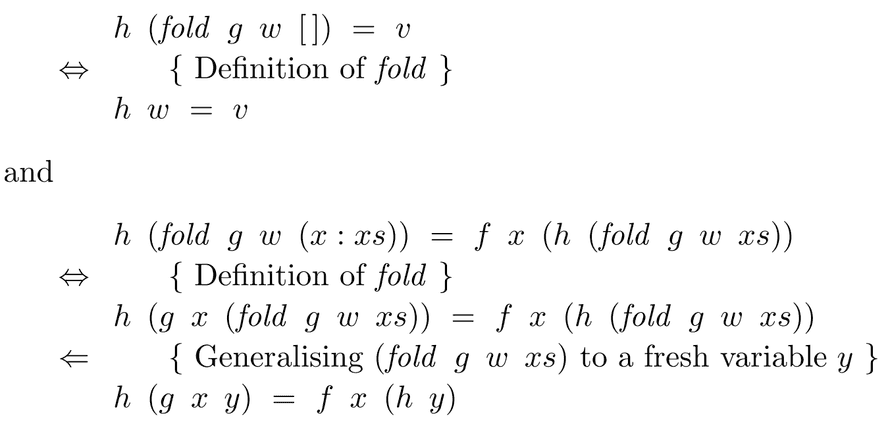To get:

Which basically says that, if the conditions on the left are met, then our composition of an arbitrary function and fold behaves exactly as a fold would.

Now we can greatly simplify our proof of the sum equation:

``````(+1) . sum = fold (+) 1
=>
(+1) . fold (+) 0 = fold (+) 1
``````

Which matches the fusion property and we're done. Simple :)

We can also use universality as a definition principle to help us transform explicit recursion into folds.

Let's take a recursively defined sum:

We want to rewrite this into a fold. That is, we're going to solve the equation `sum = fold f v` for function `f` and value `v`. Because it matches the right-hand side of the universal property, we turn it into two equations: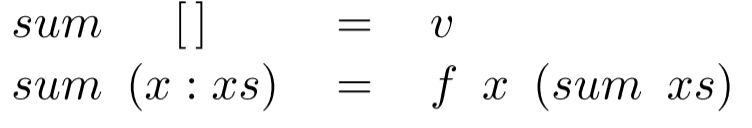We can see from the first equation that `v = 0`. From the second equation we calculate a definition for `f`.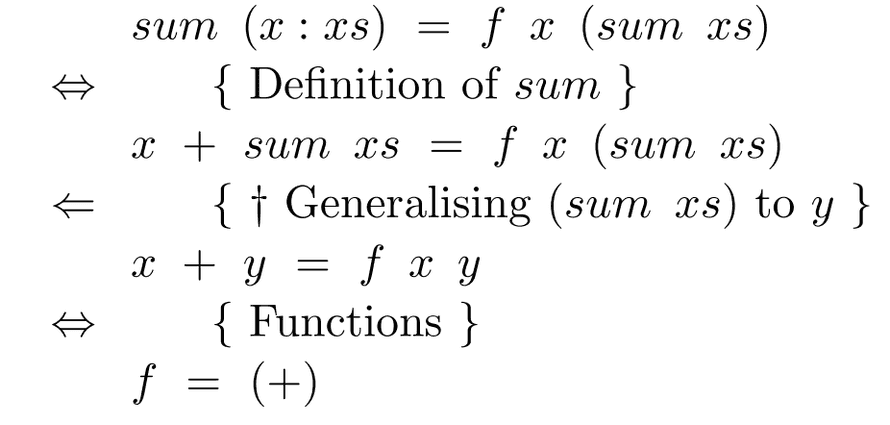We calculated that `f = (+)` and `sum = fold (+) 0`, which is a contrived example because the definition using fold is obvious, but does a good job of showing the idea behind what we're doing.

The key step (†) above is the generalisation of `sum xs` to a fresh variable `y`. This step will feature in most such calculations and isn't specific to sum.

Using this process, you can even write map as a fold:

``````map f = fold (λx ys → f x : ys) []
``````

## Increasing the power of fold with tuples

We can make fold even more powerful by using it to generate tuples. Say you want a sumlength function that returns a list's length as well as its sum:

``````sumlength   :: [Int] → (Int, Int)
sumlength xs = (sum xs, length xs)
``````

Turning this function into a single fold is more efficient because it only makes a single pass over the list.

``````sumlength = fold (λn (x, y) → (n+x, 1+y)) (0, 0)
``````

In fact, any pair of applications of fold to the same list can be rewritten as a single fold. Even a function such as dropWhile, which drops elements from a list as long as a predicate holds true.

First we need a sister function, dropWhile', that pairs the argument list together with the result:

Which, as usual, we decide is equivalent to these two equations according to the universal property: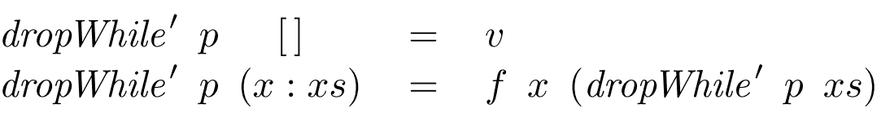It's obvious from the first equation that `v = ([], [])`. We get the definition for `f` from a calculation like this: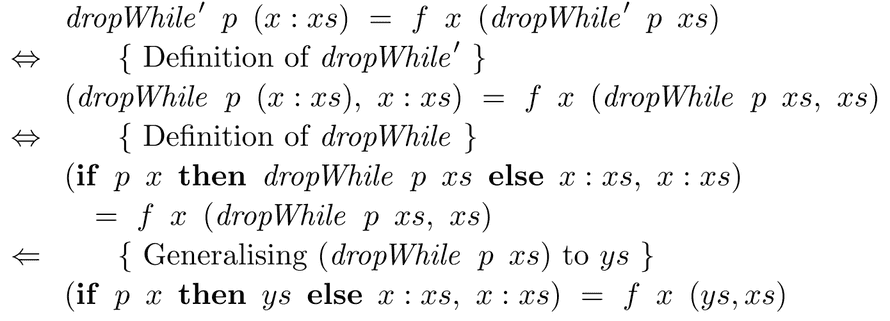And finally our `dropWhile'` function looks like this:

Composing this with `fst` gives us the normal dropWhile because the changed list ends up in the first part of the resulting tuple. `dropWhile p = fst . dropWhile' p`

This tupling technique is so powerful, we can redefine any primitive recursion function in terms of fold. The approach is usually to make a sister function that tuples the result together with the original list and work on that.

As far as I understand it, the idea is to essentially carry an extra argument into the fold so things are easier to work with. Which makes me wonder whether there's a monadic solution to this that produces cleaner code.

The paper says that if you have a function like this: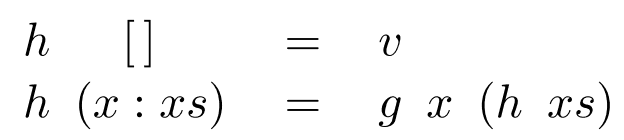You can fold this, by first introducing an extra argument, `y`, that is processed by a new function `f` in the base case, but generally passed through without change. Then you redefine `h y` with fold and introduce `xs` as an extra argument to `g`.

This gives you primitive recursion, which isn't foldable unless you use the tupling technique.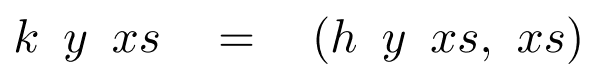And after the same sort of shenanigans as before, our function turns into a fold:

Which lets us say that `h y = fst . k y`, which is supposedly better than the definition we had before. I guess it is because we wanted to use fold and now we are.

## Using fold to generate functions

Using a language with first-order functions lets us take the power of fold further still - we can generate functions. Similar to how you'd normally replace conses with functions, you can replace them with compositions.

But that's not very interesting. I'm going to skip over the paper's definitions of various suml and foldl and such functions and go straight to the interesting example - Ackermann's function.

Ackermann's function is the first known example of a function that is computable, but isn't primitively recursive.

It's been converted to operate on lists rather than natural numbers so that n is represented as a list of n elements. The only reason we can do this, is that we're working with a higher order language.

By appealing to the universal property, we find that `ack = fold f v` is equivalent to these two equations: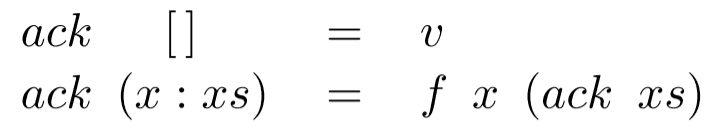A simple calculation tells us that `v = (1:)`, but we can't use the second equation the same way as before. We must first tackle `ack (x:xs)` on the left-hand side.

Now the first equation tells us that `w = ack xs` and we normally apply the universal property on the second equation to get this: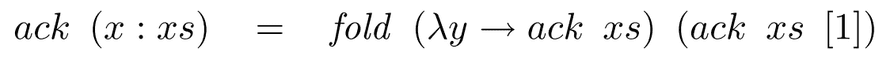We can now calculate the definition of `f`, which gives us the final folded ackerman's function: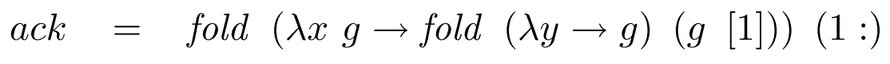It took using the universal property twice, but we did it. We defined ackerman's function as a fold.

## Fin

The paper finishes with a survey of references for even more magical things you can do with folds. Using them for datatypes other than lists, performing monadic folds, redefining fold on relations, and how helpful fold can be in automatic program transformation.

But all of that are topics for another day, today let's just agree that fold is awesome and we should all be using it much more.Published on February 13th, 2014 in 52papers52weeks, Learning, Personal, Papers

### Senior Mindset Book

Get promoted, earn a bigger salary, work for top companies

Have a burning question that you think I can answer? Hit me up on twitter and I'll do my best.

Who am I and who do I help? I'm Swizec Teller and I turn coders into engineers with "Raw and honest from the heart!" writing. No bullshit. Real insights into the career and skills of a modern software engineer.

Want to become a true senior engineer? Take ownership, have autonomy, and be a force multiplier on your team. The Senior Engineer Mindset ebook can help 👉 swizec.com/senior-mindset. These are the shifts in mindset that unlocked my career.

Curious about Serverless and the modern backend? Check out Serverless Handbook, for frontend engineers 👉 ServerlessHandbook.dev

Want to Stop copy pasting D3 examples and create data visualizations of your own? Learn how to build scalable dataviz React components your whole team can understand with React for Data Visualization

Want to get my best emails on JavaScript, React, Serverless, Fullstack Web, or Indie Hacking? Check out swizec.com/collections

Did someone amazing share this letter with you? Wonderful! You can sign up for my weekly letters for software engineers on their path to greatness, here: swizec.com/blog

Want to brush up on your modern JavaScript syntax? Check out my interactive cheatsheet: es6cheatsheet.com

By the way, just in case no one has told you it yet today: I love and appreciate you for who you are ❤️

Created by Swizec with ❤️